Latest Banking jobs   »

# Quantitative Aptitude Quiz For IBPS RRB PO Prelims 2022- 1sth July

Directions (1-5): The following pie chart shows the distribution (in degrees) of number of openings in six different, A, B, C, D, E & F, departments issued by a tech firm X. Study the data carefully and answer the following question.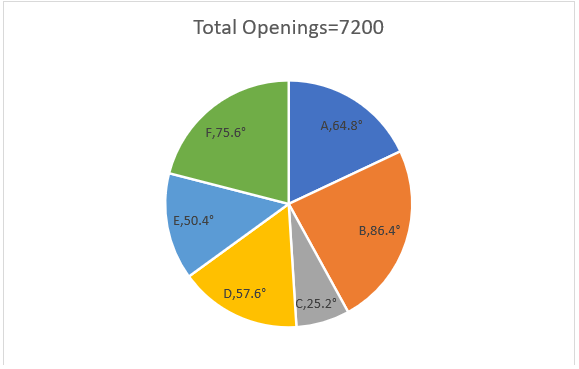Q1. Find the number of openings in Department ‘B’ is what percent of average number of openings in Department ‘A’ and ‘E’ together?
(a) 25%
(b) 150%
(c) 75%
(d) 100%
(e) 50%

Q2. Find the total number of openings in Department ‘D’ and ‘F’ together?
(a) 2712
(b) 2390
(c) 2456
(d) 2664
(e) 2558

Q3. In Department E, openings for females are 20% less then openings for males then find total number of openings for females in Department ‘E’?
(a) 362
(b) 384
(c) 522
(d) 498
(e) 448

Q4. Find the respective ratio of total number of openings in Department ‘B’ and ‘D’ together to total number of openings in Department ‘E’ and ‘F’ together?
(a) 8 : 7
(b) 4 : 7
(c) 6 : 7
(d) 9 : 7
(e) 12 : 7

Q5. Find the average number of openings in Department ‘C’, ‘E’ and ‘F’ together?
(a) 888
(b) 982
(c) 1102
(d) 1008
(e) 946

Directions (6-10): Line chart given below shows number of five different, A, B, C, D & E, types of articles sold by three different, K, L & M, sellers. Study the data carefully & answer the following.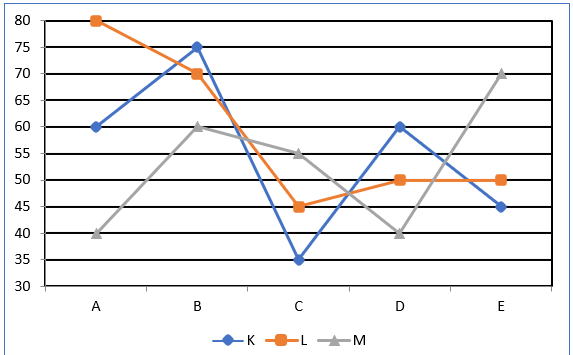Q6. Find the sum of number of article A, B and D sold by K is what percent of the article D sold by all the sellers together?
(a) 120%
(b) 130%
(c) 140%
(d) 125%
(e) 135%

Q7. Find the ratio of article C sold by all three sellers together to article E sold by all three sellers together?
(a) 7 : 10
(b) 8 : 11
(c) 9 : 11
(d) 3 : 4
(e) 9 : 13

Q8. Find the average number of articles sold by L is how much more than average number of articles sold by K?
(a) 10
(b) 8
(c) 5
(d) 6
(e) 4

Q9. If another article ‘F’ sold was 20% more than the article ‘E’ and also the ratio of article F sold by seller K, L & M was in the ratio 6:7:5 respectively, then find the number of article F sold by seller M?
(a) 45
(b) 55
(c) 30
(d) 25
(e) 60

Q10. If Price of article A is Rs. 2 while price of article B is Rs.3, then find total revenue earned by M is how much more/Less then total revenue earned by K by selling articles A and B together?
(a) Rs.95
(b) Rs.105
(c) Rs.100
(d) Rs.85
(e) Rs.90

Directions (11-15): Study the following bar graph and answer the question.
Given below is the quantities of rice, wheat and sugar (in kg) consumed by 5 different, P, Q, R, S & T, families in year 2019.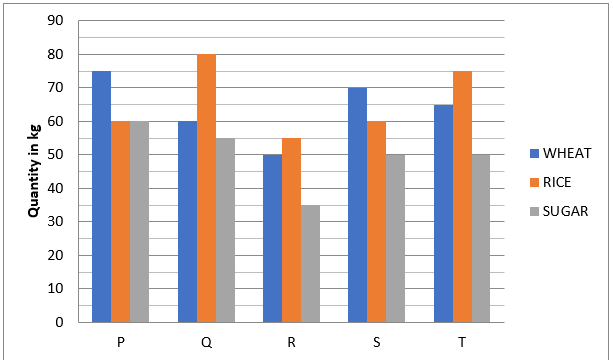Q11. What is the ratio of total quantity of rice & wheat together consumed by family R to the total quantity of wheat consumed by family T?
(a) 13 : 21
(b) 20 :13
(c) 22 : 29
(d) 21 : 13
(e) 13 : 20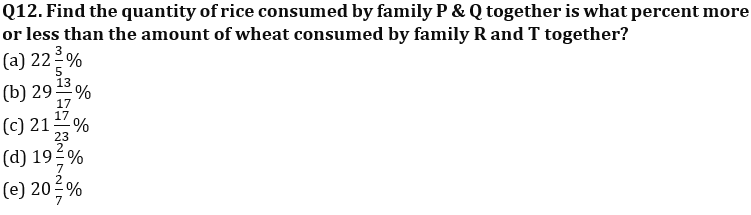Q13. Which quantity out of the three quantities consumed by all the 5 families together is maximum?
(a) Wheat
(b) Rice
(c) Sugar
(d) Both a & b
(e) None of these

Q14. In year 2020, if total quantity of wheat and rice consumed by all the family together increases by 12.5% and 33⅓% respectively, then in 2020 what is the difference between total quantity of wheat & rice consumed by all families together?
(a) 120 kg
(b) 60 kg
(c) 40 kg
(d) 100 kg
(e) 80 kg

Q15. If the amount (Rs/kg) of wheat, rice and sugar are in the ratio of 5:4:3 and the total amount paid by family Q is Rs. 12560, then find the amount paid for sugar (in Rs.)?
(a) 2640
(b) 2820
(c) 2440
(d) 2510
(e) None of these

Solutions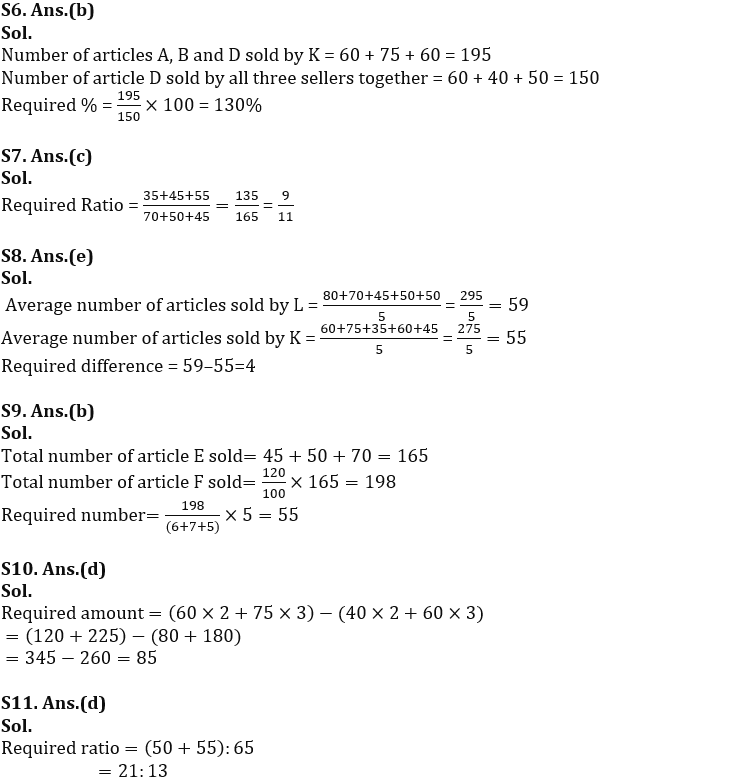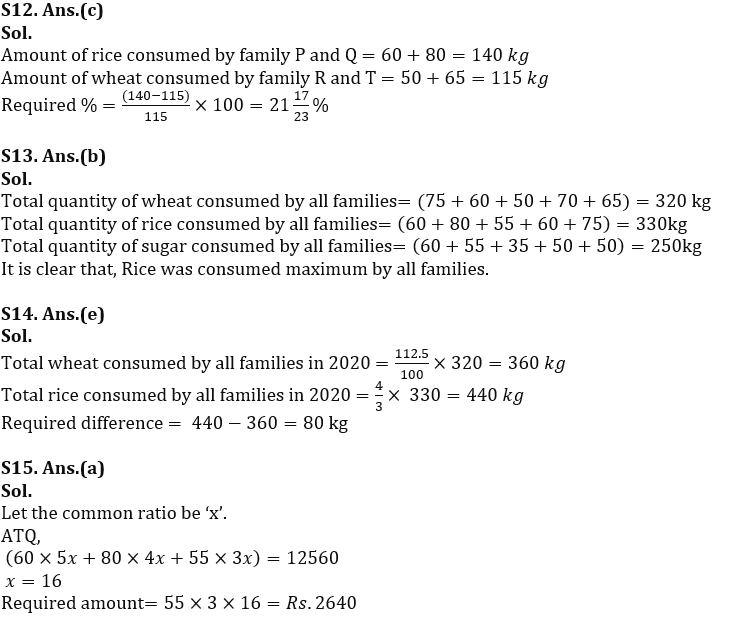#### Congratulations!Incorrect details? Fill the form again here

•Reasoning Ability Quiz For IBPS RRB PO C...
•Quantitative Aptitude Quiz For IBPS RRB ...
•Reasoning Ability Quiz For IBPS RRB PO C...
•Quantitative Aptitude Quiz For IBPS RRB ...
•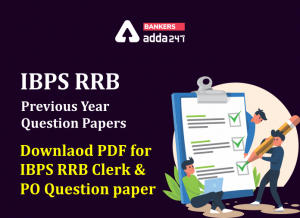IBPS RRB Previous Year Question Paper PD...
•Quantitative Aptitude Quiz For IBPS RRB ...